# Python之Numpy扩展包学习与使用——数组数据处理

Numpy是一个高性能科学计算与数据分析的Python软件包，也是很多其他Python包（如pandas、matplotlib等）的依赖。目前，Numpy是开源项目，在众多开发者的支持下，它的功能在不断扩展。详细介绍可参考Numpy官网——https://numpy.org/

### 文章目录

import numpy as np

## 1️⃣ ndarray(数组)对象

Numpy的核心是ndarray（N-dimensional array）对象，即数组对象，用于表达多维结构的数据，由相同类型的元素所构成。

``````import numpy as np

L = [1, 3, 2, 6]
a = np.array(L)
print(a.ndim)
print(a.shape)
print(a.size)
print(a.dtype)
``````

``````b = a.astype('float')
print(b.dtype)
``````

## 2️⃣ 创建数组

### 🔘1.根据已有序列数据创建数组

dtype属性用于在创建时指定数组的元素类型。

``````L = [[1, 2, 3, 4]]
a = np.array(L)

L = [, , , ]
a = np.array(L, dtype="f")
``````

[En]

The element of an array can also be a structure (object), that is, it can contain many different types of values. An array with an element type of structure is called a structure array. A structured array is similar to a table in a database, and an element in the array can be thought of as a record in the table.

[En]

Structural elements are represented by tuples, and lists of multiple tuples form an one-dimensional structural array (similar to multiple record values of multiple fields

``````c = {"names": ["city_name", "lon", "lat", "pop"], "formats": ["S32", "f", "f", "i"]}
c_type = np.dtype(c)
cities = [("beijing", 116.37, 39.92, 21707000),
("shanghai", 121.53, 31.26, 24183300),
("guangzhou", 113.25, 23.13, 14498400)]
a = np.array(cities, dtype=c_type)
print(a)
'''输出：
[(b'beijing', 116.37, 39.92, 21707000)
(b'shanghai', 121.53, 31.26, 24183300)
(b'guangzhou', 113.25, 23.13, 14498400)]'''
print(a["pop"])
'''输出：[21707000 24183300 14498400]'''
print(a["city_name"])
'''输出：
b'beijing' '''
``````

### 🔘2.创建由规则数据组成的数组

Numpy中的arange()函数和linspace()函数用于产生由规则数据组成的一维数组，两者都是按照 等间隔产生数据，前者产生为整数，后者产生为浮点数。

``````a = np.arange(1, 10, 1)
print(a)

a = np.linspace(1, 10, 5, endpoint=True)
print(a)
``````

### 🔘3.创建由随机数据组成的数组

``````a1 = np.random.rand(5)
print(a1)
a1 = np.random.rand(5, 5)

a2 = np.random.randn(5)
print(a2)

a3 = np.random.randint(low=1, high=10, size=5)
print(a3)

a4 = np.random.normal(loc=0, scale=1, size=5)
print(a4)

a4 = np.random.permutation([2, 8, 3, 4, 1])
print(a4)
``````

### 🔘4.创建特定数组

Numpy提供以下函数用于创建特定数组：
（1）ones()函数，根据指定形状和dtype创建一个全是1的数组

``````a = np.ones((3, 3), dtype='int')
'''输出：
[[1 1 1]
[1 1 1]
[1 1 1]]'''
``````

ones_like()函数，以另一个数组为参数（包括形状和dtype）创建一个全是1的数组

``````a = np.random.rand(3, 3)
b = np.ones_like(a)
print(b)
'''输出：
[[1. 1. 1.]
[1. 1. 1.]
[1. 1. 1.]]'''
``````

（2）zeros()函数，根据指定形状和dtype创建一个全是0的数组

``````b = np.zeros((3, 3))
print(b)
'''输出：
[[0. 0. 0.]
[0. 0. 0.]
[0. 0. 0.]]'''
``````

zeros_like()函数，以另一个数组为参数（包括形状和dtype）创建一个全是0的数组

``````a = np.random.rand(3, 3)
b = np.zeros_like(a)
print(b)
'''输出：
[[0. 0. 0.]
[0. 0. 0.]
[0. 0. 0.]]'''
``````

【→👆→（1）和（2）中的函数生成的数组的 默认类型都是float64。】

（3）empty()函数，根据指定形状和dtype创建一个元素全为空的数组

``````b = np.empty((3, 3))
``````

empty_like()函数，以另一个数组为参数（包括形状和dtype）创建一个元素全为空的数组

``````a = np.random.rand(3, 3)
b = np.empty_like(a)
``````

``````[[0.00000000e+000 0.00000000e+000 0.00000000e+000]
[0.00000000e+000 0.00000000e+000 2.52961611e-321]
[1.69115935e-306 8.91238232e-313 1.44635573e-307]]
``````

（4）eye(N, M=None)函数，创建一个二维的单位数组（对角线为1，其余全为0）

``````b = np.eye(N=3, M=3)
print(b)
'''输出：
[[1. 0. 0.]
[0. 1. 0.]
[0. 0. 1.]]'''
``````

## 3️⃣改变数组形状

### 🔘1.通过shape属性值改变

``````a = np.array([[1, 2, 3, 4], [5, 6, 7, 8], [9, 10, 11, 12]])
print(a.shape)
a.shape = 4, 3
print(a.shape)
a.shape = 2, 2, -1
print(a.shape)
``````

### 🔘2.通过数组对象方法改变

（1） reshape()方法，改变数组为新的形状，如下：

``````a = np.array([[1, 2, 3, 4], [5, 6, 7, 8], [9, 10, 11, 12]])
print(a.shape)
a_reshape = a.reshape(1, 3, -1)
print(a_reshape.shape)
print(a_reshape)
'''输出：
[[[ 1  2  3  4]
[ 5  6  7  8]
[ 9 10 11 12]]]'''
``````

（2） transpose()方法，数组转置（类似矩阵的转置），如下：

``````a = np.array([[1, 2, 3, 4], [5, 6, 7, 8], [9, 10, 11, 12]])
print(a.shape)
print(a)
'''输出：
[[ 1  2  3  4]
[ 5  6  7  8]
[ 9 10 11 12]]'''
a_transpose = a.transpose()
print(a_transpose.shape)
print(a_transpose)
'''输出：
[[ 1  5  9]
[ 2  6 10]
[ 3  7 11]
[ 4  8 12]]'''
``````

``````a_transpose1 = a_transpose.transpose(1, 0)
print(a_transpose1)
'''输出：
[[ 1  2  3  4]
[ 5  6  7  8]
[ 9 10 11 12]]'''
``````

（3） flatten()方法，用于将数组展平（变为一维数组），如下：

``````a = np.array([[1, 2, 3, 4], [5, 6, 7, 8], [9, 10, 11, 12]])
a_flatten = a.flatten()
print(a_flatten)
'''输出：
[ 1  2  3  4  5  6  7  8  9 10 11 12]'''
``````

(4) squeeze()方法，用于去除数组中长度为1的轴。如果想要 指定轴（axis=i），则指去除指定的长度为1的轴（如长度不为1，则会保错）

``````a = np.array([[[1, 2, 3, 4], [5, 6, 7, 8], [9, 10, 11, 12]]])
a_squeeze = a.squeeze()
print(a_squeeze)
'''输出：
[[ 1  2  3  4]
[ 5  6  7  8]
[ 9 10 11 12]]'''
``````

## 4️⃣数组的索引和切片

[En]

The indexes and slices of an array are used to return an element or a range of elements in an array, or to reassign an element or a range of elements in an array.

### 🔘1.数组的索引操作

``````a = np.array([[1, 2, 3, 4], [5, 6, 7, 8], [9, 10, 11, 12]])
print(a[1, 2])
print(a)
``````

### 🔘2.数组的切片操作

``````a = np.array([[1, 2, 3, 4], [5, 6, 7, 8], [9, 10, 11, 12]])
print(a[0:2])
print(a[0:2, 0:2])
``````

[En]

Note: the slicing operation returns the referenced data, and the modifications to the sliced data will be reflected in the original data (similarly, the modifications to the original data will also affect the sliced data), as follows:

``````a = np.array([[1, 2, 3, 4], [5, 6, 7, 8], [9, 10, 11, 12]])
print(a[0, 0])
b = a[0, 0:2]
print(b)
b = 0
print(a[0, 0])
``````

## 5️⃣数组的连接与切分

### 🔘1.数组的连接操作

（数组的连接是把多个数组连接到一起产生一个新的数组）

（1） vstack()函数 或 row_stack()函数，用于垂直方向连接

``````a = np.zeros((3, 3))
b = np.ones((3, 3))
print(np.vstack((a, b)).shape)
``````

（2） hstack()函数 或 column_stack()函数，用于水平方向连接

``````a = np.zeros((3, 3))
b = np.ones((3, 3))
print(np.hstack((a, b)).shape)
``````

：column_stack()函数与hstack()函数，在对一维数组的连接操作有一定的区别，column_stack()函数得到一个二维数据，hstack()函数还是一维数组，如下：

``````a = np.zeros((3,))
b = np.ones((3,))
print(np.hstack((a, b)))
'''输出：[0. 0. 0. 1. 1. 1.]'''

print(np.column_stack((a, b)))
'''输出：
[[0. 1.]
[0. 1.]
[0. 1.]]'''
``````

### 🔘2.数组的切分操作

（数组的切分是数组连接的逆操作）

（1） hsplit()函数，用于沿水平方向对数组进行均匀切分

``````a = np.array([[1, 2, 3, 4], [5, 6, 7, 8], [9, 10, 11, 12]])
b, c = np.hsplit(a, 2)
print(b)
'''输出：
[[ 1  2]
[ 5  6]
[ 9 10]]'''
print(c)
'''输出：
[[ 3  4]
[ 7  8]
[11 12]]'''
``````

（2） vsplit()函数，用于沿垂直方向对数组进行均匀切分

``````a = np.array([[1, 2, 3, 4], [5, 6, 7, 8], [9, 10, 11, 12]])
b, c, d = np.vsplit(a, 3)
print(b)
'''输出：
[[1 2 3 4]]'''
print(c)
'''输出：
[[5 6 7 8]]'''
print(d)
'''输出：
[[ 9 10 11 12]]'''
``````

## 6️⃣数组的运算

### 🔘1.基本数学运算

[En]

Arrays can perform basic mathematical operations such as addition, subtraction, multiplication and division, which can be operations between data or between arrays and scalars.

``````a = np.array([[1, 2, 3, 4], [5, 6, 7, 8], [9, 10, 11, 12]])
b = np.array([[12, 11, 10, 9], [8, 7, 6, 5], [4, 3, 2, 1]])
print(a + b)
'''输出：
[[13 13 13 13]
[13 13 13 13]
[13 13 13 13]]'''
print(a + 1)
'''输出：
[[ 2  3  4  5]
[ 6  7  8  9]
[10 11 12 13]]'''
c = np.array([10, 20, 30, 40])
print(a + c)
'''输出：
[[11 22 33 44]
[15 26 37 48]
[19 30 41 52]]'''
``````

### 🔘2.数学函数运算

Numpy中常用的数学函数如下（图源网络）：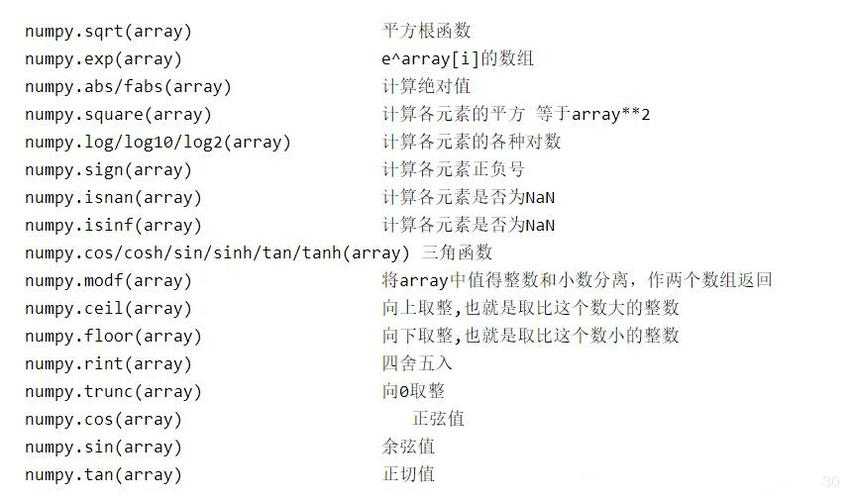### ; 🔘3.比较和逻辑运算

（1） 数组之间及数组和标量之间可以进行>、>=、

``````a = np.array([[1, 2, 3, 4], [5, 6, 7, 8], [9, 10, 11, 12]])
b = a > 5
print(b)
'''输出：
[[False False False False]
[False  True  True  True]
[ True  True  True  True]]'''
``````

（2） 布尔型数组可以进行&（和）、|（或）、^（非）等逻辑运算，返回的也是布尔类型的数组。

``````a = np.array([[1, 2, 3, 4], [5, 6, 7, 8], [9, 10, 11, 12]])
b = (a > 4) & (a < 8)
print(b)
'''输出：
[[False False False False]
[ True  True  True False]
[False False False False]]'''
``````

（3） 如果要对数组按照某个条件表达式进行判断后重新赋值，可利用where函数，函数形式为：where(cond, xarr, yarr)

``````a = np.array([[1, 2, 3, 4], [5, 6, 7, 8], [9, 10, 11, 12]])
print(np.where(a > 6, 1, 0))
'''输出：
[[0 0 0 0]
[0 0 1 1]
[1 1 1 1]]'''
``````

### 🔘4.线性代数运算

Numpy中的linalg模块提供大量的线性代数函数（基于Fortran库），可对数组进行线性代数运算。
numpy.linalg模块中常用函数（图源网络）：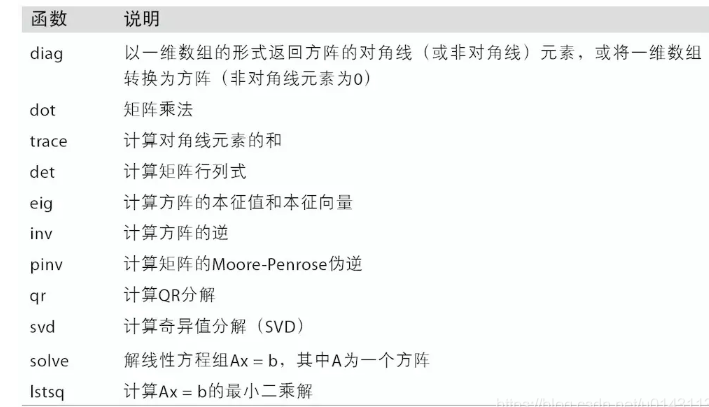``````import numpy as np
from numpy import linalg
a = np.array([[1, 2], [3, 4]])
print(linalg.inv(a))
'''输出（逆矩阵）：
[[-2.   1. ]
[ 1.5 -0.5]]'''
``````

## 6️⃣数组元素的统计与排序

### 🔘1.数组元素的统计

（1） sum()，对数组中全部或某轴向的元素求和

``````a = np.array([[1, 2, 3, 4], [5, 6, 7, 8], [9, 10, 11, 12]])
print(a.sum())
``````

（2） mean()，计算数组的算数平均值

``````a = np.array([[1, 2, 3, 4], [5, 6, 7, 8], [9, 10, 11, 12]])
print(a.mean())
``````

（3） std()、var()，计算数组标准差、方差

``````a = np.array([[1, 2, 3, 4], [5, 6, 7, 8], [9, 10, 11, 12]])
print(a.std())
print(a.var())
``````

（4） max()、min()，计算数组的最大值、最小值

``````a = np.array([[1, 2, 3, 4], [5, 6, 7, 8], [9, 10, 11, 12]])
print(a.max())
print(a.min())
``````

（5） argmax()、argmin()，获取数组最大值、最小值的下标

``````a = np.array([[1, 2, 3, 4], [5, 6, 7, 8], [9, 10, 11, 12]])
print(a.argmax())
print(a.argmin())
``````

（6） ......

``````a = np.array([[1, 2, 3, 4], [5, 6, 7, 8], [9, 10, 11, 12]])
print(np.sum(a, axis=0))
print(np.sum(a, axis=1))
``````

### 🔘2.数组元素的排序

（1） sort()函数，返回一个按从小到大顺序进行排序并包含原数组中的所有元素的新数组

``````a = np.array([3, 3, 1, 2, 6, 1, 6, 9, 8])
print(np.sort(a))
``````

（2） unique()函数，返回一个按从小到大顺序进行排序并只包含原数组中不同值的元素的新数组

``````a = np.array([3, 3, 1, 2, 6, 1, 6, 9, 8])
print(np.unique(a))
``````

Original: https://blog.csdn.net/m0_53156691/article/details/123439815
Author: 蔺WIT
Title: Python之Numpy扩展包学习与使用——数组数据处理

# （二）安装 pytorch

## 2.1 创建虚拟环境``````conda create --name pytorch python=3.8.1
``````

``````activate pytorch
``````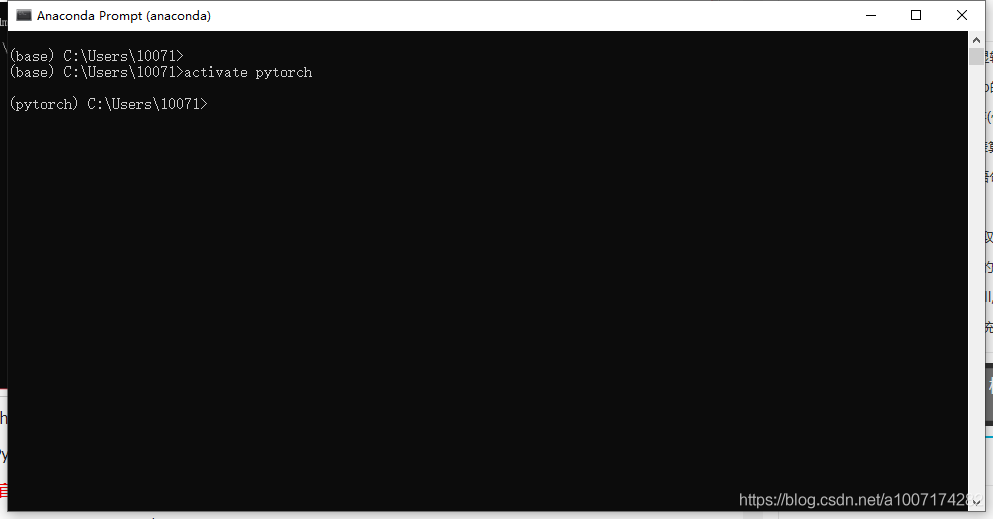## 2.2正式安装pytorch

****：pytorch 官网链接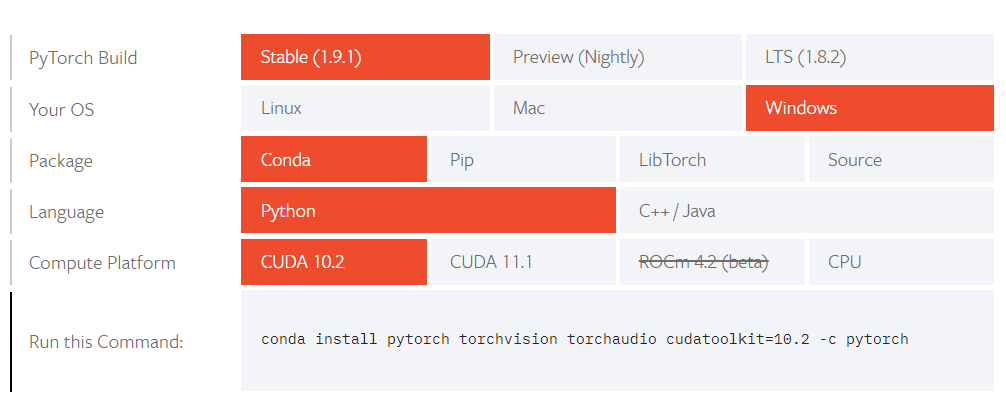• 本人采用的方法
在pytorch虚拟环境下，创建.condarc文件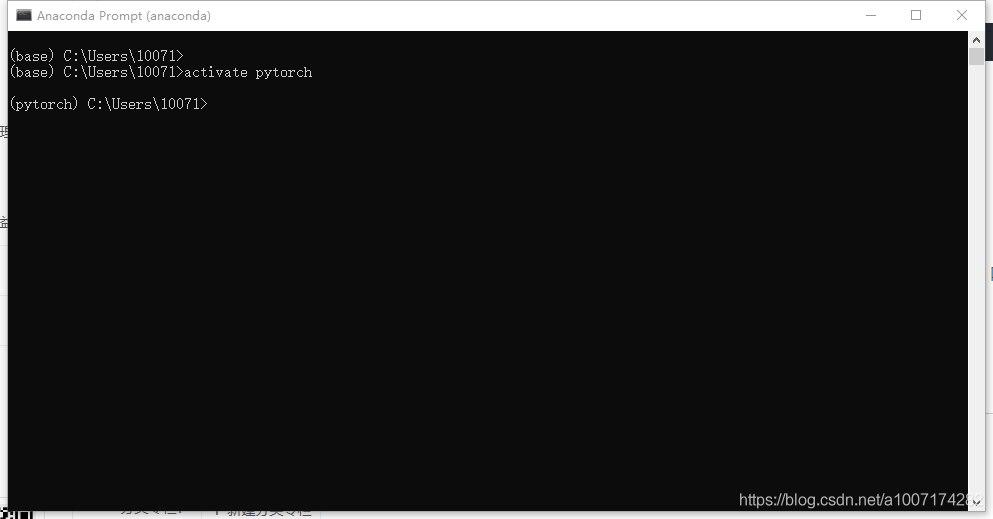在虚拟环境中输入如下命令：
``````conda config --set show_channel_urls yes
``````
• 之后在自己电脑的用户文件下找到一个.condarc文件用记事本打开这个文件，然后用如下代码代替其中的内容：
``````channels:
- defaults
show_channel_urls: true
channel_alias: https://mirrors.tuna.tsinghua.edu.cn/anaconda
default_channels:
- https://mirrors.tuna.tsinghua.edu.cn/anaconda/pkgs/main
- https://mirrors.tuna.tsinghua.edu.cn/anaconda/pkgs/free
- https://mirrors.tuna.tsinghua.edu.cn/anaconda/pkgs/r
- https://mirrors.tuna.tsinghua.edu.cn/anaconda/pkgs/pro
- https://mirrors.tuna.tsinghua.edu.cn/anaconda/pkgs/msys2
custom_channels:
conda-forge: https://mirrors.tuna.tsinghua.edu.cn/anaconda/cloud
msys2: https://mirrors.tuna.tsinghua.edu.cn/anaconda/cloud
bioconda: https://mirrors.tuna.tsinghua.edu.cn/anaconda/cloud
menpo: https://mirrors.tuna.tsinghua.edu.cn/anaconda/cloud
pytorch: https://mirrors.tuna.tsinghua.edu.cn/anaconda/cloud
simpleitk: https://mirrors.tuna.tsinghua.edu.cn/anaconda/cloud
``````

`&#x8BB0;&#x4F4F;.condarc&#x914D;&#x7F6E;&#x597D;&#x540E;&#x4E00;&#x5B9A;&#x8981;&#x4FDD;&#x5B58;&#x3002;`

• 或者使用清华镜像源
在浏览pytorch安装帮助的相关帖子时有人说清华源停止镜像了，但是现在清华源已经恢复提供镜像了，所以还是可以用的。附上清华源Anaconda镜像使用帮助，大家可以读一下这段帮助（不读也没关系下面给出详细步骤）。
``````conda install pytorch torchvision torchaudio cudatoolkit=10.2 -c pytorch
``````

## 2.3 验证是否安装成功

• 一个是在prompt里面

（1）在命令行左边为 pytorch 环境中，输入 python

（2）之后，输入 import torch，如果没有报错，意味着 PyTorch 已经顺利安装了。

• 一个是在jupyter notebook里面调用
首先，在菜单中打开Anaconda Prompt，然后安装插件。
命令行输入：
``````conda install nb_conda
``````

``````conda install ipykernel
``````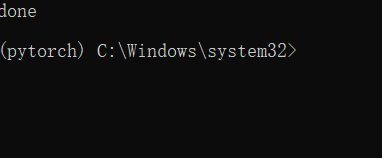# （三）本文参考链接如下：（感谢各位大佬）

Original: https://blog.csdn.net/weixin_54546190/article/details/120754242
Author: ☞源仔
Title: pytorch安装详细步骤